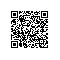# 前言

*#_*
_*__
_#_@

# 正文

## 问题分析

开始

当队列为非空时，继续执行，否则算法结束
出队列取得队列头顶点V；访问并标记为已访问
查找列头顶点V的所有邻接顶点W1，W2，...Wn
对于上述的所有每一个顶点Wn
循环
若Wn在close list中， 则继续遍历下一个顶点Wn+1
否则将Wn入队列，并加入到close list中

## 代码

public class Point {
private int X;
private int Y;

public Point(int x, int y){
this.X = x;
this.Y = y;
}

public int getX() {
return X;
}
public void setX(int x) {
X = x;
}
public int getY() {
return Y;
}
public void setY(int y) {
Y = y;
}

//判断两个点是否坐标相同
public static boolean isSamePoint(Point point1, Point point2){
if(point1.getX() == point2.getX() && point1.getY() == point2.getY())
return true;
return false;
}

}

import java.util.ArrayList;
import java.util.List;

public class State {
//机器人位置
private Point robotLocation;

//操作,分为N(向上移动一格), S(向下移动一格), W(向左移动一格), E(向右移动一格)以及C(清理灰尘)
private String operation;

//当前节点的父节点, 用于达到目标后进行回溯
private State previousState;

//灰尘所在坐标的list
private List<Point> dirtList;

public Point getRobotLocation() {
return robotLocation;
}

public void setRobotLocation(Point robotLocation) {
this.robotLocation = robotLocation;
}

public String getOperation() {
return operation;
}

public void setOperation(String operation) {
this.operation = operation;
}

public State getPreviousState() {
return previousState;
}

public void setPreviousState(State previousState) {
this.previousState = previousState;
}

public List<Point> getDirtList() {
return dirtList;
}

public void setDirtList(List<Point> dirtList) {
this.dirtList = new ArrayList<Point>();
for(Point point : dirtList){
this.dirtList.add(point);
}
}

//用于判断两个节点是否相同
public static boolean isSameState(State state1, State state2){
//若机器人位置不同,则节点不同
if(!Point.isSamePoint(state1.getRobotLocation(), state2.getRobotLocation()))
return false;
//若灰尘列表长度不同, 则节点不同
else if(state1.getDirtList().size() != state2.getDirtList().size())
return false;
//若前两者都相同, 则判断两个state中的灰尘列表中的灰尘坐标是否完全相同
else{
for(Point point : state1.getDirtList())
{
boolean same = false;
for(Point point2 : state2.getDirtList())
{
if(Point.isSamePoint(point, point2))
same = true;
}
if(!same)
return false;
}
}
//若满足上述所有条件, 则两节点相同。
return true;
}

}


import java.util.ArrayList;
import java.util.LinkedList;
import java.util.List;
import java.util.Queue;
import java.util.Scanner;
import java.util.Stack;

public class Robot {
//行数
public static int colomnNum;

//列数
public static int rowNum;

//障碍物数量
public static int obstacleNum;

//用于深度优先搜索的栈
public static Stack<State> stack;

//用于广度优先搜索的队列
public static Queue<State> queue;

//地图
public static String[][] map;

//灰尘坐标列表
public static List<Point> dirtList;

//closeList，用于存放已经存在的state
public static List<State> closeList;

//遍历总耗费
public static int cost = 0;

public static void main(String[] args) {
State initialState = new State();
Scanner sc = new Scanner(System.in);
System.out.println("Please Enter Row Number:");
rowNum = sc.nextInt();
System.out.println("Please Enter Colomn Number:");
colomnNum = sc.nextInt();
map = new String[rowNum][colomnNum];
dirtList = new ArrayList<Point>();
closeList = new ArrayList<State>();
sc.nextLine();
for(int i=0; i<rowNum; i++)
{
System.out.println("Please Enter the Elements in row " + (i + 1) + ":");
String line = sc.nextLine();
for(int j=0; j<colomnNum; j++)
{
//统计障碍物数量
if(line.charAt(j) == '#')
{
obstacleNum++;
}

//将灰尘格子坐标存入list中
if(line.charAt(j) == '*')
{
dirtList.add(new Point(i, j));
}

//设置机器人初始坐标
if(line.charAt(j) == '@')
{
initialState.setRobotLocation(new Point(i, j));
}

//初始化地图
map[i][j] = line.charAt(j) + "";
}
}
sc.close();
initialState.setDirtList(dirtList);

//初始化栈
stack = new Stack<State>();

//初始化队列
queue = new LinkedList<State>();
//stack.push(initialState);
closeList.add(initialState);
queue.add(initialState);
cost++;

//遍历开始
while(!queue.isEmpty()){
//State state = stack.pop();

//取出队列中第一个state
State state = queue.poll();

//如果达到目标,输出结果并退出
if(isgoal(state)){
output(state);
return;
}
calculate(state);
}
}

public static void calculate(State state){
//获取当前机器人的坐标
int x = state.getRobotLocation().getX();
int y = state.getRobotLocation().getY();

//如果当前的点是灰尘并且没有被清理
if(map[x][y].equals("*") && !isCleared(new Point(x, y), state.getDirtList())){
State newState = new State();
List<Point> newdirtList = new ArrayList<Point>();
//在新的state中,将灰尘列表更新,即去掉当前点的坐标
for(Point point : state.getDirtList())
{
if(point.getX() == x && point.getY() == y)
continue;
else
newdirtList.add(new Point(point.getX(), point.getY()));
}
newState.setDirtList(newdirtList);
newState.setRobotLocation(new Point(x, y));
//C代表Clean操作
newState.setOperation("C");
newState.setPreviousState(state);

//若新产生的状态与任意一个遍历过的状态都不同,则进入队列
if(!isDuplicated(newState)){
//stack.push(newState);
queue.add(newState);
closeList.add(newState);
cost++;
}
}

//若当前机器人坐标下方有格子并且不是障碍物
if(x + 1 < rowNum)
{
if(!map[x+1][y].equals("#"))
{
State newState = new State();
newState.setDirtList(state.getDirtList());
newState.setRobotLocation(new Point(x + 1, y));
//S代表South,即向下方移动一个格子
newState.setOperation("S");
newState.setPreviousState(state);
if(!isDuplicated(newState)){
//stack.push(newState);
queue.add(newState);
//加入到closeList中
closeList.add(newState);
cost++;
}
}
}

//若当前机器人坐标上方有格子并且不是障碍物
if(x - 1 >= 0)
{
if(!map[x-1][y].equals("#"))
{
State newState = new State();
newState.setDirtList(state.getDirtList());
newState.setRobotLocation(new Point(x - 1, y));
//N代表North,即向上方移动一个格子
newState.setOperation("N");
newState.setPreviousState(state);
if(!isDuplicated(newState)){
//stack.push(newState);
queue.add(newState);
closeList.add(newState);
cost++;
}
}
}

//若当前机器人坐标左侧有格子并且不是障碍物
if(y - 1 >= 0)
{
if(!map[x][y-1].equals("#"))
{
State newState = new State();
newState.setDirtList(state.getDirtList());
newState.setRobotLocation(new Point(x, y - 1));
//W代表West,即向左侧移动一个格子
newState.setOperation("W");
newState.setPreviousState(state);
if(!isDuplicated(newState)){
//stack.push(newState);
queue.add(newState);
closeList.add(newState);
cost++;
}
}
}

//若当前机器人坐标右侧有格子并且不是障碍物
if(y + 1 < colomnNum)
{
if(!map[x][y+1].equals("#"))
{
State newState = new State();
newState.setDirtList(state.getDirtList());
newState.setRobotLocation(new Point(x, y + 1));
//E代表East,即向右侧移动一个格子
newState.setOperation("E");
newState.setPreviousState(state);
if(!isDuplicated(newState)){
//stack.push(newState);
queue.add(newState);
closeList.add(newState);
cost++;
}
}
}

}

//判断是否已经达到目标,即当前遍历到的state中手否已经没有灰尘需要清理
public static boolean isgoal(State state){
if(state.getDirtList().isEmpty())
return true;
return false;
}

//输出,由最后一个state一步一步回溯到起始state
public static void output(State state){
String output = "";
//回溯期间把每一个state的操作(由于直接输出的话是倒序)加入到output字符串之前,再输出output
while(state != null){
if(state.getOperation() != null)
output = state.getOperation() + "\r\n" + output;
state = state.getPreviousState();
}
System.out.println(output);
//最后输出遍历过的节点(state)数量
System.out.println(cost);
}

//判断节点是否存在,即将state与closeList中的state相比较,若都不相同则为全新节点
public static boolean isDuplicated(State state){
for(State state2 : closeList){
if(State.isSameState(state, state2))
return true;
}
return false;
}

//判断地图中当前位置的灰尘在这个state中是否已经被除去。
public static boolean isCleared(Point point, List<Point> list){
for(Point p : list){
if(Point.isSamePoint(p, point))
return false;
}
return true;
}

}


Please Enter Row Number:
3
Please Enter Colomn Number:
3
Please Enter the Elements in row 1:
@#*
Please Enter the Elements in row 2:
*__
Please Enter the Elements in row 3:
#*_
S
C
E
S
C
N
E
N
C

45

@#*
*__
#*_

45是程序遍历过的节点（state）数量。

*#_*
_*__
_#_@

Please Enter Row Number:
3
Please Enter Colomn Number:
4
Please Enter the Elements in row 1:
*#_*
Please Enter the Elements in row 2:
_*__
Please Enter the Elements in row 3:
_#_@
N
N
C
S
W
W
C
W
N
C

56

56是程序遍历过的节点（state）数量。

O(BM)BM

# 结束语使用钉钉扫一扫加入圈子
+ 订阅